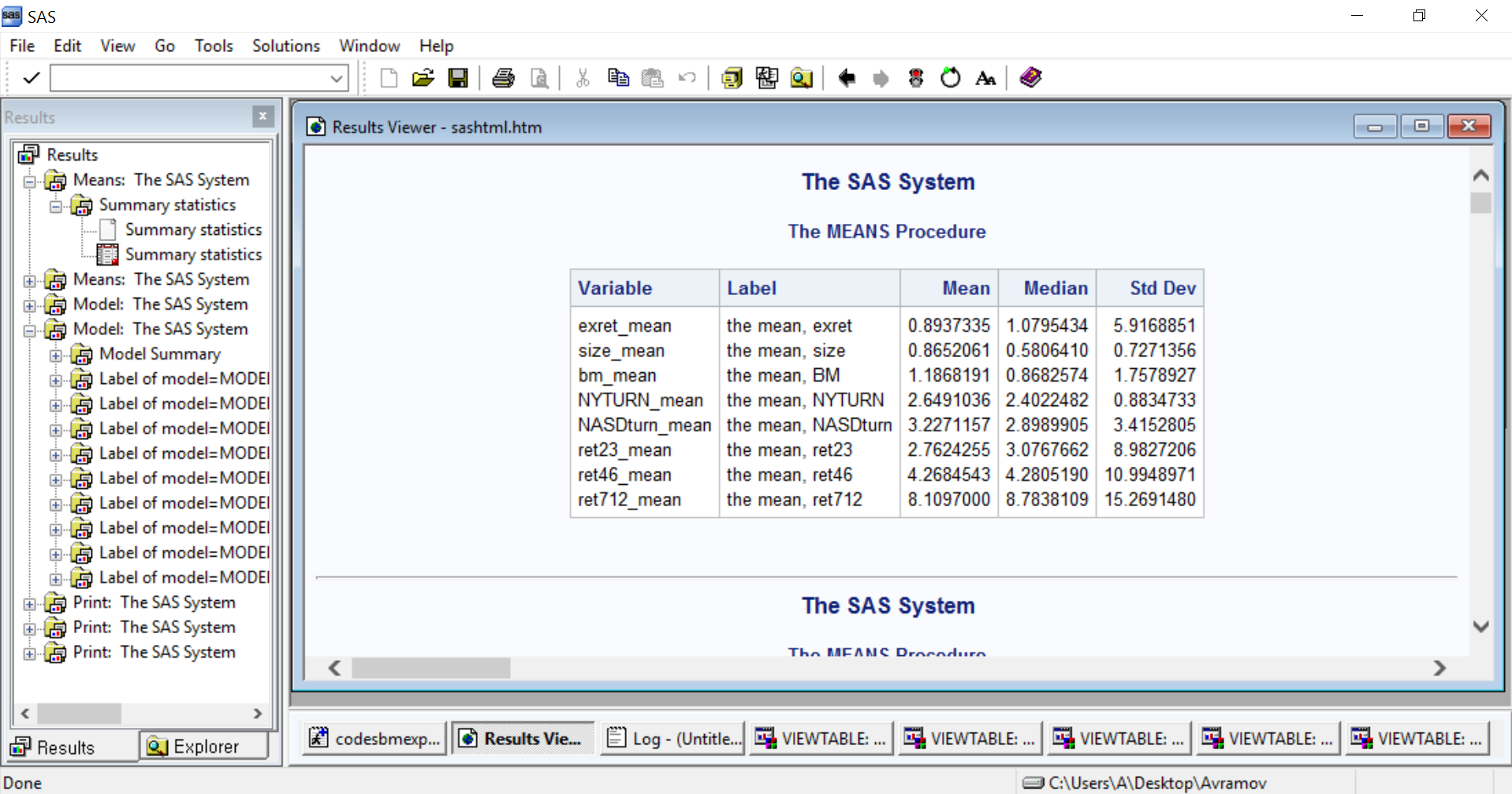## Transposing Statistics from PROC MEANS

After running my codes SAS ODS generate very nice output which looks like this:

[ODS Output in Result Viewer]This is the table I actually want to use futher to merge with other variables, But SAS file created in Explorer along with this output, looks like this.

[SAS File]

 exret_mean the mean, exret 0.893734 1.07954 5.91689 size_mean the mean, size 0.865206 0.580641 0.727136 bm_mean the mean, BM 1.18682 0.868257 1.75789 NYTURN_mean the mean, NYTURN 2.6491 2.40225 0.883473 NASDturn_mean the mean, NASDturn 3.22712 2.89899 3.41528 ret23_mean the mean, ret23 2.76243 3.07677 8.98272 ret46_mean the mean, ret46 4.26845 4.28052 10.9949 ret712_mean the mean, ret712 8.1097 8.78381 15.2691

I am trying to take transpose of above SAS file, to construct a SAS file similar ODS output table. I tried these codes

``````proc transpose data=NYNASD out=NYNASD3
name=Variable prefix=mean;
run;``````

But the problem is PROC TRANSPOSE creates an output where mean median and std dev are transposed in each seperate row like this.

[SAS File created after transpose]

Obs    Variable                _LABEL_           mean1

1    NASDturn_mean_Mean      Mean       3.2271156602
2    NASDturn_mean_Median    Median     2.8989904612
3    NASDturn_mean_StdDev    Std Dev    3.4152804673
4    NYTURN_mean_Mean        Mean       2.6491035766
5    NYTURN_mean_Median      Median     2.4022482113
6    NYTURN_mean_StdDev      Std Dev    0.8834733073
7    bm_mean_Mean            Mean       1.1868190703
8    bm_mean_Median          Median     0.8682573682
9    bm_mean_StdDev          Std Dev    1.7578926815
10    exret_mean_Mean         Mean       0.8937335333
11    exret_mean_Median       Median     1.0795434232
12    exret_mean_StdDev       Std Dev    5.9168850852
13    ret23_mean_Mean         Mean       2.7624255427
14    ret23_mean_Median       Median     3.0767662175
15    ret23_mean_StdDev       Std Dev    8.9827206345
16    ret46_mean_Mean         Mean       4.2684542516
17    ret46_mean_Median       Median     4.2805190421
18    ret46_mean_StdDev       Std Dev    10.994897056
19    ret712_mean_Mean        Mean       8.1097000264
20    ret712_mean_Median      Median      8.783810874
21    ret712_mean_StdDev      Std Dev    15.269148018
22    size_mean_Mean          Mean       0.8652060691
23    size_mean_Median        Median     0.5806409775
24    size_mean_StdDev        Std Dev    0.7271356297

I WANT

1) Ideally a way to directly convert ODS Table in result viewer to SAS work file in explorer (without exporting to excel and importing again)

2) To modify transpose codes so, mean median & std dev of each variable is reported in one row, just like they were created in SAS ODS output

1 ACCEPTED SOLUTION

Accepted Solutionsart297
Opal | Level 21

## Re: Transposing Statistics

Here is a blog that does (I think) precisely what you want: http://blogs.sas.com/content/sgf/2015/07/17/customizing-output-from-proc-means/

Art, CEO, AnalystFinder.com

Editor's note: including final solution.

STACKODSOUTPUT work like a charm. Here are the rewriten codes with stackodsoutput option so others can get benefit too.

Just add stackoutput option in the step which is generating required ODS output. This will stack statistics of same variables togather in SAS file same as in ODS output file

``````proc means data=NYNASD_CS1 stackodsoutput mean median std;
var  exret size bm NYturn NASDturn ret23 ret46 ret712;
ods output summary= Summ_NYNASD;
run;``````

5 REPLIES 5art297
Opal | Level 21

## Re: Transposing Statistics

Here is a blog that does (I think) precisely what you want: http://blogs.sas.com/content/sgf/2015/07/17/customizing-output-from-proc-means/

Art, CEO, AnalystFinder.com

Editor's note: including final solution.

STACKODSOUTPUT work like a charm. Here are the rewriten codes with stackodsoutput option so others can get benefit too.

Just add stackoutput option in the step which is generating required ODS output. This will stack statistics of same variables togather in SAS file same as in ODS output file

``````proc means data=NYNASD_CS1 stackodsoutput mean median std;
var  exret size bm NYturn NASDturn ret23 ret46 ret712;
ods output summary= Summ_NYNASD;
run;``````

## Re: Transposing Statistics

If you do read the blog post, read all the way to the end.

The STACKODS option pretty much gives you a nice clean table.

## Re: Transposing Statistics

Thank you very much  @art297 and @Reeza . I could'nt imagine that I spend so much time to try doing this when the solution was so easy.

STACKODSOUTPUT work like a charm. Here are the rewriten codes with stackodsoutput option so others can get benefit too.

Just add stackoutput option in the step which is generating required ODS output. This will stack statistics of same variables togather in SAS file same as in ODS output file

``````proc means data=NYNASD_CS1 stackodsoutput mean median std;
var  exret size bm NYturn NASDturn ret23 ret46 ret712;
ods output summary= Summ_NYNASD;
run;``````

Once again thanks for the contribution

## Re: Transposing Statistics

PROC MEANS with the STACKODSOUTPUT option produces many statistics. However, there are a few descriptive statistics that are not available in PROC MEANS. The UNIVARIATE procedure provides additional statistics. If you use PROC UNIVARIATE, then you can use the OUTTABLE= option to generate 46 different descriptive statistics.

For complete details, see the article "Save descriptive statistics for multiple variables in a SAS data set." Here is a brief example from the blog post:

``````proc univariate data=sashelp.cars outtable=UniSummary NORMAL noprint;
run;            /* omit VAR statement to analyze all numerical variables */

proc print data=UniSummary label;
var _var_ _label_ _NObs_ _Mean_ _Std_ _Min_ _Max_;  /* MANY more stats available! */
run;``````

## Re: Transposing Statistics

Thanks a lot @Rick . I will also try using UNIVARIATE and see the results
Discussion stats
• 5 replies
• 5925 views
• 4 likes
• 4 in conversation